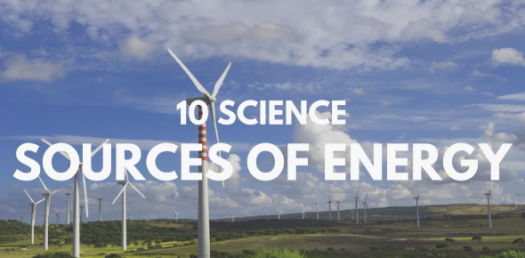# Quiz: Sources Of Energy

15 Questions | Attempts: 1020SettingsThere are different sources of energy that people get to use on a daily basis. Some of the ways used to harness this energy affect the environment in a negative way where others don’t. What is your understanding of some of the sources of energy and its importance? Take up this test and get to find out. All the best!

• 1.
A good fuel is one that possesses:
• A.

High calorific value and low ignition temperature

• B.

Low calorific value and low ignition temperature

• C.

High calorific value and moderate ignition temperature

• D.

Low calorific value and moderate ignition temperature

• 2.
There are four fuels which all contain only carbon and hydrogen. The fuel having highest calorific value will be one which has:
• A.

More of carbon but less of hydrogen

• B.

Less of carbon but more of hydrogen

• C.

Equal proportions of carbon and hydrogen

• D.

Less of carbon as well as less of hydrogen

• 3.
Coke is more valuable when used:
• A.

As a fuel for industrial boilers

• B.

As an oxidizing agent

• C.

As a reducing agent

• D.

As a fuel in domestic ovens

• 4.
In a hydroelectric power plant more electrical power can be generated if water falls from a greater height because:
• A.

Its temperature increases

• B.

A larger amount of potential energy is converted into kinetic energy

• C.

The electricity content water increases with height

• D.

More water molecules dissociate into ions

• 5.
In order to make efficient solar cooker, the cover of the cooker box should be made of:
• A.

Transparent plastic sheet

• B.

Shining aluminium sheet

• C.

Butter paper sheet

• D.

Transparent glass sheet

• 6.
If solar constant is 1.4 kW/m2, then the solar energy received by 1 m2 area in one hour is:
• A.

5040 J

• B.

54.0 kJ

• C.

5040 kJ

• D.

504.0 J

• 7.
The rise of sea-water during high tide is caused by the gravitational pull of the:
• A.

Sun

• B.

Earth

• C.

Moon

• D.

Mars

• 8.
One atomic mass unit (u) is equivalent to an energy of:
• A.

93.1 MeV

• B.

9.31 MeV

• C.

1 MeV

• D.

931 MeV

• 9.
The energy produced by converting 1 gram mass of nuclear fuel into energy completely is:
• A.

Option 1

• B.

9 ×1014 J

• C.

9 ×1015 J

• D.

9 ×1013 J

• 10.
The disposal of wastes produced in a nuclear power plant poses a big problem because it is:
• A.

• B.

Highly inflammable

• C.

Extremely foul smelling

• D.

Too heavy

• 11.
The energy-efficient device for producing light is:
• A.

DLF

• B.

CFL

• C.

FCL

• D.

LPG

• 12.
The radiation in the sunlight that gives us the feeling of hotness is:
• A.

• B.

Infra-red

• C.

Red

• D.

Ultra-violet

• 13.
Which of the following fuels would be used for a barbecue?
• A.

Charcoal

• B.

Coke

• C.

Oil

• D.

Coal

• 14.
The diagram summarizes four stages for a way of producing electricity. Which of the following matches stage 2?
• A.

Turn Generator

• B.

Hot volcanic rocks

• C.

Rotating turbine

• D.

Making steam flow fast

• 15.
The diagram shows a simple calorimeter system for measuring the heat given out by a liquid fuel burner. The experiment data for four fuels A, B, C, and D are given below. 100 ml (100g) of water was used in the calorimeter to 'collect' the heat given out. The mass of fuel burned and the temperature rise of the water is given. From the data which fuel is the LEAST efficient in terms of energy released per unit mass?
• A.

Mass of fuel = 0.80g, temperature rise 12oC

• B.

Mass of fuel = 1.20 g, temperature rise 24oC

• C.

Mass of fuel = 0.50 g, temperature rise 9oC

• D.

Mass of fuel = 1.75g, temperature rise 28oC

## Related TopicsBack to top
×

Wait!
Here's an interesting quiz for you.